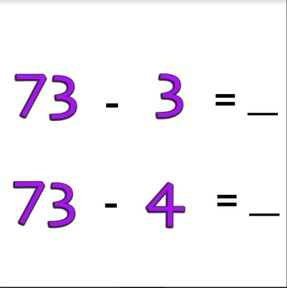Subtraction to 100 via one more/one less

# Subtraction to 100 via one more/one less8,000 schools use Gynzy92,000 teachers use Gynzy1,600,000 students use Gynzy

## General

Students learn to subtract numbers to 100 by adding one more or taking one away.

2.NBT.B.5

## Relevance

Discuss with students that it is useful to be able to subtract with one more or one less. If you can determine when you need to add one more or take one away, you can solve sets of subtraction problems.

## Introduction

Show students an image of stairs and ask students what the correct order for the given numbers is when you set them in order from smallest to largest. Set the numbers on their places on the stairs. Then give each student a number card. Students must find one or more neighbor numbers to their number and then stand in the correct order. Students should end in groups of 3. Make sure that you skip a number after every three, so you don't end up with a group of 6 students who are all each other's neighbor numbers.

## Development

Show the MAB blocks on the interactive whiteboard. Say that there are 80 blocks in total and 40 are taken away. The difference is 40. Discuss the other subtraction problems shown by the blocks. Ask students if they notice anything about the subtraction problems. The second number (the subtrahend) is one larger in each problem. Sometimes there aren't images/objects that can be counted with a math problem. Sometimes there are only numbers. Compare both subtraction problems. If the subtrahend is one more in the second subtraction problem, then the difference is one less in the second problem. Show the problems on the interactive whiteboard and ask students to determine the differences. Ask them how they determined the difference for the second and third problems, did they solve the problems completely or did they just count one more?
Next show students the blocks again and show them that there are 67 blocks in total and that you take 50 away. The difference is 17. Discuss the rest of the subtraction problems and ask students if they notice anything about the subtraction problems. Tell students that the subtrahend is one less each time. Sometimes there are only numbers. Compare both subtraction problems. If the minuend is one less in the second problem, then the answer is also one less. Show the problems on the interactive whiteboard and ask students to determine the differences. Ask them how they determined the difference for the second and third problems, did they solve the problems completely or did they just count one more?
Tell students that when the minuend in the second problem is one less than the minuend in the first problem, that the difference is one more. Practice the subtraction problems shown and ask students what their strategy was. Show the problems on the interactive whiteboard and ask students to find the differences. Ask them to write down the answers on a sheet of paper/mini whiteboard, and to hold them up to check their work.

Check that students are able to subtract with numbers to 100 via one more/less by asking the following questions:
- What do you look at to determine if you need to add one/take one away?
- Calculate the following problems: 79 - 9 = and 79 - 10 =
- Calculate the following problems: 46 - 6 = and 46 - 5 =

## Guided practice

Students are first given subtraction problems with one more/less consisting of sets of two subtraction problems. Then they are given sets of three subtraction problems to solve using one more/less.

## Closing

Discuss with students that it is important to be able to subtract numbers to 100 via one more/less so you can quickly solve sets of calculations without needing to recalculate each subtraction problem. Next show the subtraction problems on the interactive whiteboard and ask students to solve them individually or in pairs. Discuss the answers and ask students to explain their strategy. Ask if they recalculated each problem or if they were able to solve via one more/less.

## Teaching tips

Students who have difficulty with this learning goal can be supported by the use of manipulatives like MAB blocks. Have them set out the total, and then add one more block, or take one block away.

## Instruction materials

Optional: MAB blocks or other manipulatives

### The online teaching platform for interactive whiteboards and displays in schools

• Save time building lessons

• Manage the classroom more efficiently

• Increase student engagement